# 一类带时间窗的公共租赁自行车调度模型Dispatching Model of Public Rental Bicycle with Time Windows

DOI: 10.12677/OJTT.2020.93021, PDF, HTML, XML, 下载: 61  浏览: 104  国家自然科学基金支持

Abstract: In order to solve the problem of unbalanced space-time distribution of bikes in public bike rental points, four mixed integer linear programming models with time windows were established in the case of single and multiple dispatching centers by adopting two ideas of loading before unloading, and loading and unloading at the same time. The model aims at the minimum total delivery miles, where some constraints such as the supply and demand relationship of the lease point and the rated capacity of the truck are considered. Finally, Cplex is used to solve the exact solution of an example, where the truck distribution route is given, and the scheme differences of the four models are compared, so as to verify the correctness of the model.

1. 引言

2. 问题描述和数学模型

2.1. 假设

(1) 与既有智能调度平台数据交互，收集所有租赁点在各个时间段的缺少或多余自行车数；

(2) 所有供应点和需求点均在卡车配送路径中，并且只被允许一辆车访问；

(3) 忽略卡车在租赁点的装卸自行车时间对配送线路的影响。

2.2. 符号

$i,j$ ：租赁点或调度中心；

$k$ ：车辆。

$N$ ：租赁点集合；

$O$ ：调度中心集合；

$K$ ：车辆集合。

${p}_{j}$ ：租赁点j的自行车需求量，若 ${p}_{j}>0$，则多余；否则，缺少；

${z}_{j}$ ：租赁点j的供需标识，若 ${p}_{j}>0$，则 ${z}_{j}=1$ ；否则， ${z}_{j}=-1$

${d}_{ij}$ ：公共自行车租赁点i和j之间距离；

${q}_{i}^{k}$ ：车辆k访问租赁点i后的自行车数量，即断面流量；

${t}_{i}^{k}$ ：车辆k访问租赁点i的时间；

$\left[{l}_{i},{u}_{i}\right]$ ：租赁点i的时间窗；

$C$ ：车辆的最大容量；

$D$ ：车辆的最大里程；

$M$ ：一个比较大的固定值。

${x}_{ij}^{k}$ ：车辆k依次访问租赁点i和j；

${y}_{i}^{k}$ ：车辆k是否访问租赁点i；

${p}_{j}^{k}$ ：车辆k访问租赁点j时的装卸自行车数；

Uik：辅助变量。

2.3. 目标函数

$\mathrm{min}\underset{\forall k\in K}{\sum }\underset{\forall i\in N\cup O}{\sum }\underset{\forall j\in N\cup O}{\sum }{x}_{ij}^{k}{d}_{ij}$ (1)

2.4. 约束条件

$\underset{\forall i\in N}{\sum }{y}_{i}^{k}\ge 1\text{ }\forall k\in K$ (2)

$\underset{\forall k\in K}{\sum }{y}_{i}^{k}=1\text{ }\forall i\in N\cup O\text{ }\forall k\in K$ (3)

$\underset{\forall i\in N}{\sum }{x}_{ij}^{k}=\underset{\forall i\in N}{\sum }{x}_{ji}^{k}={y}_{i}^{k}\text{ }\forall j\in N,\forall k\in K$ (4)

$\underset{\forall i\in O}{\sum }{x}_{ij}^{k}=\underset{\forall i\in O}{\sum }{x}_{ji}^{k}=1\text{ }\forall j\in N,\forall k\in K$ (5)

${U}_{ik}-{U}_{jk}+\left(|H|*{x}_{ij}^{k}\right)\ge |H|-1,\forall i,j\in N\cup O,\forall k\in K$ (6)

${q}_{i}^{k}+{p}_{j}^{k}-\left(1-{x}_{ij}^{k}\right)\cdot M\le {q}_{j}^{k}\text{ }\forall i,j\in N\cup O,\forall k\in K$ (7)

${q}_{i}^{k}+{p}_{j}^{k}+\left(1-{x}_{ij}^{k}\right)\cdot M\ge {q}_{j}^{k}\text{ }\forall i,j\in N\cup O,\forall k\in K$ (8)

${t}_{i}^{k}+{T}_{ij}-\left(1-{x}_{ij}^{k}\right)\cdot M\le {t}_{j}^{k}\text{ }\forall i,j\in N\cup O,\forall k\in K$ (9)

${t}_{i}^{k}+{T}_{ij}+\left(1-{x}_{ij}^{k}\right)\cdot M\ge {t}_{j}^{k}\text{ }\forall i,j\in N\cup O,\forall k\in K$ (10)

${l}_{i}\le {t}_{i}^{k}\le {u}_{i}\text{ }\forall i,j\in N\cup O,\forall k\in K$ (11)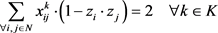(12)

$\underset{\forall j\in N}{\sum }{x}_{0j}^{k}\cdot {p}_{j}\ge 0\text{ }\forall k\in K$ (13)

$\underset{\forall j\in N}{\sum }{x}_{j0}^{k}\cdot {p}_{j}\le 0\text{ }\forall k\in K$ (14)

${p}_{j}^{k}\le {p}_{j}\text{ }\text{ }{p}_{j}>0\text{ }\forall j\in N,\forall k\in K$ (15)

${p}_{j}^{k}={p}_{j}\text{ }{p}_{j}<0\text{ }\forall j\in N,\forall k\in K$ (16)

${q}_{i}^{k}=0\text{ }\forall i\in O,\forall k\in K$ (17)

${q}_{i}^{k}\le C\text{ }\forall i\in N,\forall k\in K$ (18)

$\underset{\forall i\in N\cup O}{\sum }\underset{\forall j\in N\cup O}{\sum }{x}_{ij}^{k}{d}_{ij}\le D\text{ }\forall k\in K$ (19)

3. 算例分析Table 1. Basic information of lease points

4. 结论分析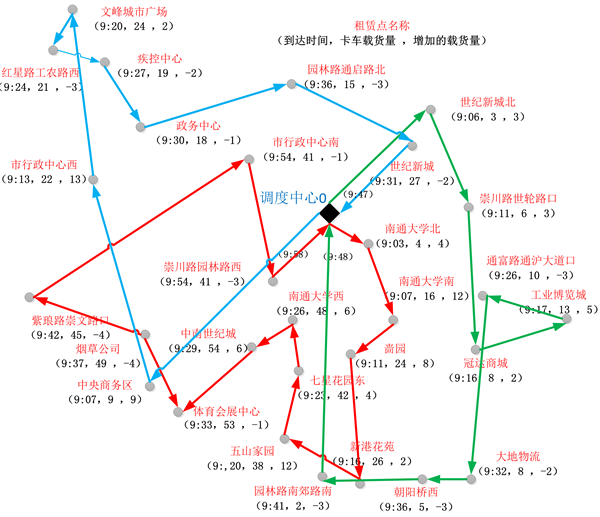Figure 1. The first dispatch and then unload distribution line of a single dispatch center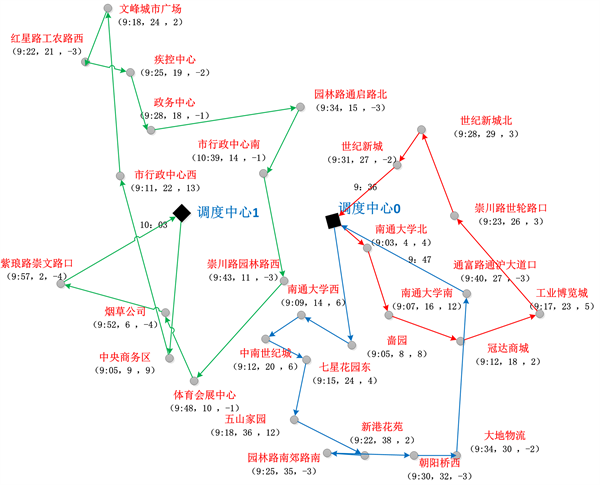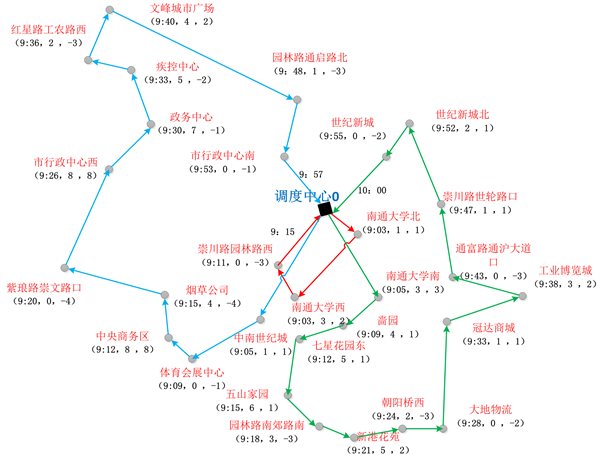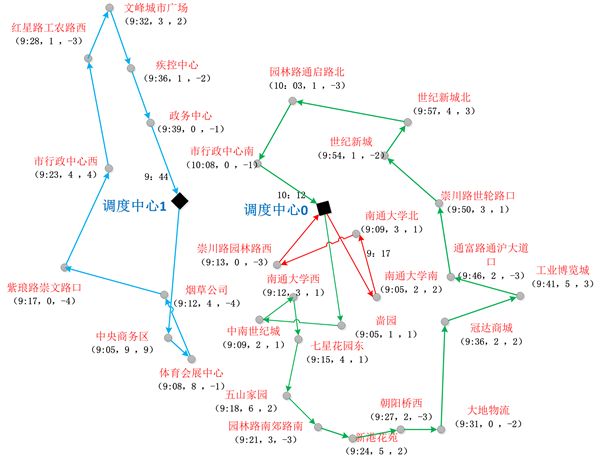Table 2. Scheme comparison of four scheduling models

NOTES

*通讯作者。

  Raviv, T., Tzur, M. and Forma, I.A. (2013) Static Repositioning in a Bike-Sharing System: Models and Solution Ap-proaches. EURO Journal on Transportation and Logistics, 2, 187-229. https://doi.org/10.1007/s13676-012-0017-6  Ho, S.C. and Szeto, W.Y. (2014) Solving a Static Repositioning Problem in Bike-Sharing Systems Using Iterated Tabu Search. Transportation Research Part E, 69, 180-198. https://doi.org/10.1016/j.tre.2014.05.017  董红召, 赵敬洋, 郭海锋, 等. 公共慢行系统的动态调度建模与滚动时域调度算法研究[J]. 公路工程, 2009(6) :43-46.  鲍娜. 城市公共自行车租赁点选址决策及调度模型研究[D]: [硕士学位论文]. 西安: 长安大学, 2012.  刘登涛, 方文道, 章坚民, 等. 公共自行车交通系统调度算法[J]. 计算机系统应用, 2011, 20(9):112-116.  张辉, 郑彭军. 基于蚁群算法的城市公共自行车调度研究[J]. 科技与管理, 2015, 17(6):32-36.  徐建闽, 秦筱然, 马莹莹. 公共自行车多层次分区调度方法研究[J]. 交通运输系统工程与信息, 2017, 17(1): 212-219.  胡列格, 夏云, 王佳, 等. 城市公共自行车高峰期需求不均衡的调度优化研究[J]. 铁道科学与工程学报, 2015(2): 441-448.  Ho, S.C. and Szeto, W.Y. (2017) A Hybrid Large Neighborhood Search for the Static Multi-Vehicle Bike-Repositioning Problem. Transportation Research Part B, 95, 340-363. https://doi.org/10.1016/j.trb.2016.11.003  Li, Y.F., Szetob, W.Y., Long, J.C. and Shui, C.S. (2016) A Multiple Type Bike Repositioning Problem. Transportation Research Part B, 90, 263-278. https://doi.org/10.1016/j.trb.2016.05.010  柳机鹏, 丁卫东, 程逸曼. 公共自行车系统站问调度优化研究[J]. 城市公共交通, 2011(1): 65-69.